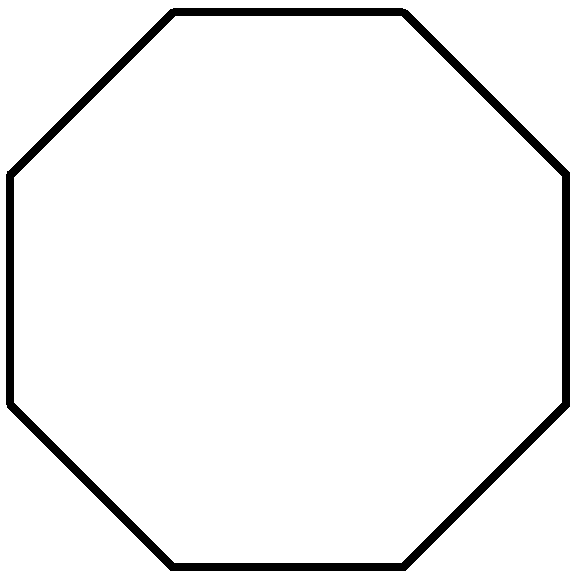Problems
226 - Polygons

Polygons

Time Limit: 1 sec

The Problem

There is a N sided polygon. How many triangles you can form from that N sided triangle ?The Input

Input will be a number (2<N<1000) denoting the number of sides of the polygon.

The Output

The output will be the amount triangle that can be formed and should be printed in a separate line.

Sample Input

5

Sample Output

3

Problem Setter: Parag Paul